﻿ Getting Started Tutorials - MechDesigner > Tutorial 18: Math FB > Step 18.4: Lissajous Figures

# Step 18.4: Lissajous Figures

### Plotting Lissajous Curves with Parametric Equations.

We only need the position equations to plot the Lissajous Curve.

These are simple parametric curves.

This topic here is only because they look nice to me!

#### Parameters Equations

 We will use parametric equations in the Math FB to calculate the X,Y coordinates of the Lissajous Curve. The parametric equations for the Lissajous Curve of curves are: x = cos(a*t) y = sin(b*t) If you change a parametric-constants (a, b), you will plot a different Lissajous Curve. Parameters and Variables a = the frequency of the motion along the X-axis b = the frequency of the motion along the Y-axis t = the independent variable: 0 to 360 (or 2*pi) Three(3) Inputs: a, b, and t Two(2) Outputs: x and y.

#### Input-Connector and Output-Connectors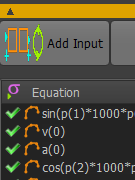Input-Connectors We need three input-connectors: three parameters (a, b) and one variable (t) 1.Double-click the Math FB to open a Math FB dialog-box 2.Click Add Input button to add three inputs to the Math FB 3.Click the Update button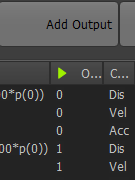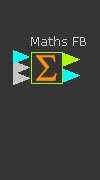Output-Connectors We need two output-connectors to give the data for the X and Y-axes 1.Double-click the Math FB to open the Math FB dialog-box 2.Click the Add Output button to add two outputs from the Math FB The units for the output-channel is dependent on the Output Data Type. 3.Set the Output Data Type to  Linear Coordinates 4.Click the Update button

#### Parametric Equations in the Math FB

 Equations for the Position (Displacement) for the two outputs. The Lissajous equations for the X and Y coordinates are given here again: •x = cos(a×t) ..... Equation 1 •y = sin(b×t) ..... Equation 2 We must re-write these for the Math FB. Each parameter or variable at the input is represented by: p(0) = t p(1) = a p(2) = b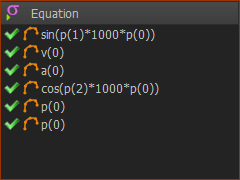1.Enter the equations very carefully - see the image to the left. •Equation 1 = cos((p(1)× 1000)× p(0)) •Equation 2 = sin((p(2)× 1000)× p(0)) 2.Click Update button to confirm changes. The output a Sin and a Cos functions each have a range of ±1 unit  (a minimum of -1 and a maximum of 1) Inside the Math FB, all parameters are converted to SI units. Thus a Range of 1 is a range of 1 meter. Outside the Math FB, the range of ±1m will be a range of ±1000mm at the X and Y-axis Piggyback Sliders.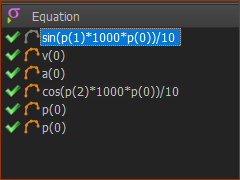Thus, we may need to scale the output to reduce its range. To Scale the Range we can use •Add Gearing FBs. For example, a Gearing Ratio of 0.1 will give a range of ±100 at the X-and Y-axis Sliders or •Edit the Equations in the Math FB. For example, we divide each equation by 10 to give a range of ±100 at the X-and Y-axis Sliders <<< We have now add /10 to the equations to give a Slider Range of 100mm

#### The Piggyback Slider Model for the Lissajous Figures

 The Top Output Connector is for the X-axis motion The Bottom Output-Connector is for the Y-axis motion. We must add two Sliders, in the Piggyback configuration. We can then •connect the Top connector to the Motion-Dimension to move the horizontal Slider •connect the Bottom connector to the Motion-Dimension to move the vertical Slider. We add Trace-Point it the n the Piggyback Slider will plot the Lissajous Figures - when the equations are correct!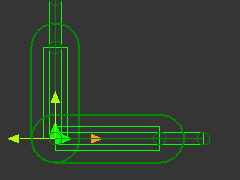The Mechanical model in MechDesigner Because we will use parametric equations for X the Y-axes, we will use Piggyback Sliders.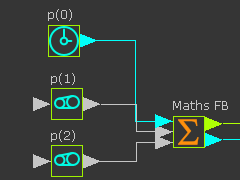The Math FB - input connectors •t .... the angle of the function from 0 to 2*pi - from the output of a Linear-Motion FB •a ... the frequency of oscillation of the X-axis - from a Gearing FB -(Add after Gearing-Ratio) •b ... the frequency of oscillation of the Y-axis - from a Gearing FB (Add after Gearing-Ratio) Note: Outside the Math FB the Range of t is 0 - 360 degrees Inside the Math FB the Range of t is 0 - 20*pi radians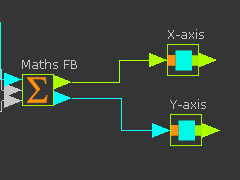Math FB - output variable connectors The Math FB has two outputs: •x ... the horizontal position of the X-Slider •y ... the vertical position of the Y-Slider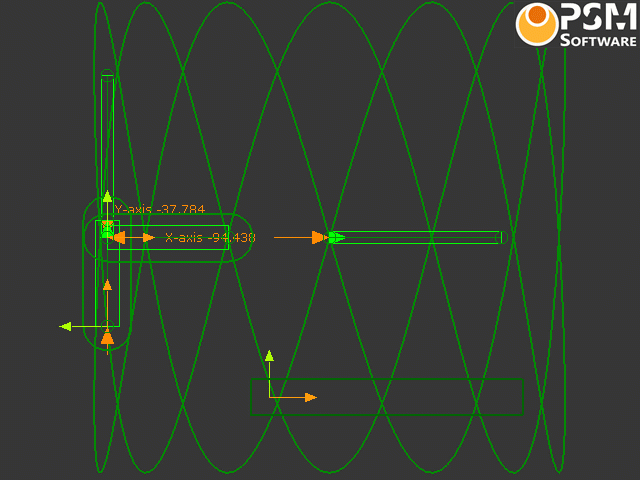Video of Lissajous Curves - Click to Play The Trace-Point of the Piggyback Slider We can add a Trace-Point to show the Path, of the Y-axis Slider -as that combines the motion of the X-axis and the Y-axis   The values of p(1) = a = 2 p(2) = b = 7